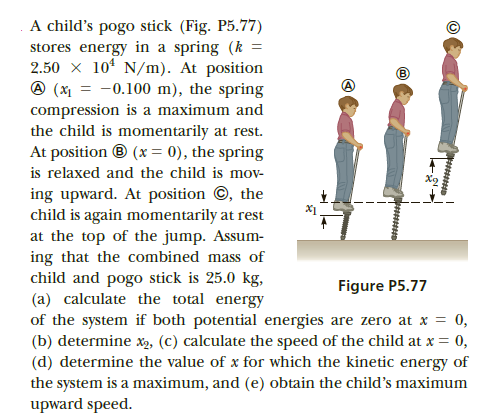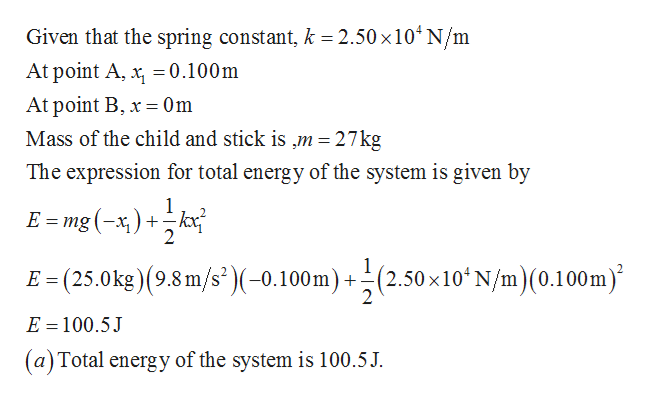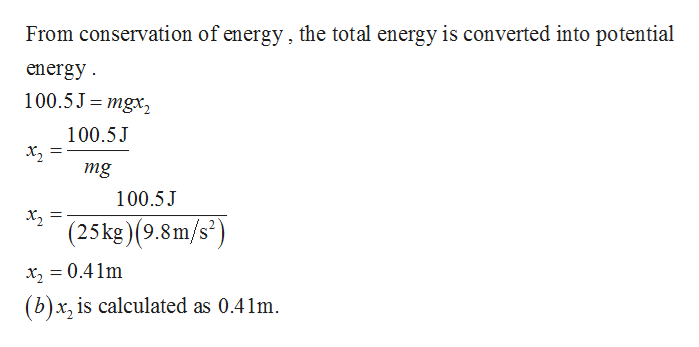# A child's pogo stick (Fig. P5.77)stores energy in a spring (k =2.50 x 10* N/m). At position@ (x = -0.100 m), the springcompression is a maximum andthe child is momentarily at rest.At position ® (x = 0), the springis relaxed and the child is mov-ing upward. At position ©, thechild is again momentarily at restat the top of the jump. Assum-ing that the combined mass ofchild and pogo stick is 25.0 kg,(a) calculate the total energyof the system if both potential energies are zero at x = 0,(b) determine x, (C) calculate the speed of the child at x = 0,(d) determine the value of x for which the kinetic energy ofthe system is a maximum, and (e) obtain the child's maximumupward speed.X1Figure P5.77

Question
38 viewshelp_outlineImage TranscriptioncloseA child's pogo stick (Fig. P5.77) stores energy in a spring (k = 2.50 x 10* N/m). At position @ (x = -0.100 m), the spring compression is a maximum and the child is momentarily at rest. At position ® (x = 0), the spring is relaxed and the child is mov- ing upward. At position ©, the child is again momentarily at rest at the top of the jump. Assum- ing that the combined mass of child and pogo stick is 25.0 kg, (a) calculate the total energy of the system if both potential energies are zero at x = 0, (b) determine x, (C) calculate the speed of the child at x = 0, (d) determine the value of x for which the kinetic energy of the system is a maximum, and (e) obtain the child's maximum upward speed. X1 Figure P5.77 fullscreen
check_circle

Step 1help_outlineImage TranscriptioncloseGiven that the spring constant, k = 2.50 x10ʻ N/m At point A, x, =0.100m At point B, x = 0m Mass of the child and stick is ,m = 27kg The expression for total energy of the system is given by kx E = mg (-x) + E = (25.0kg)(9.8 m/s²)(-0.100m) + ÷(2.50 ×10ʻN/m)(0.100m)* (2.50 ×10ʻ N/m)(0.100m) E = 100.5J (a) Total energy of the system is 100.5J. fullscreen
Step 2help_outlineImage TranscriptioncloseFrom conservation of energy , the total energy is converted into potential energy. 100.5J= mgx, 100.5J X2 mg 100.5J X, = (25kg)(9.8m/s²) x, = 0.4 1m (b)x, is calculated as 0.41m. fullscreen

### Want to see the full answer?

See Solution

#### Want to see this answer and more?

Solutions are written by subject experts who are available 24/7. Questions are typically answered within 1 hour.*

See Solution
*Response times may vary by subject and question.
Tagged in

### Work,Power and Energy